﻿ Reciprocity in Experimental Modal Analysis

### Reciprocity in Experimental Modal Analysis

Peter Pavelka, Róbert Huňady, Martin Hagara, František Trebuňa

American Journal of Mechanical Engineering

## Reciprocity in Experimental Modal Analysis

Peter Pavelka1,, Róbert Huňady1, Martin Hagara1, František Trebuňa1

1Department of Applied Mechanics and Mechanical Engineering, Faculty of Mechanical Engineering, Letná 9, 042 00 Košice, Slovakia

### Abstract

This paper deals with modal analysis and estimation of modal parameters of an analyzed structure. An aim of the paper is to verify one of four basic assumptions considered in modal testing. This property is reciprocity. Reciprocity means that the frequency response function measured between two degrees-of-freedom must be the same regardless of which degree-of-freedom is input or output. In the paper the experimental and numerical modal analysis of a cantilever beam are presented. There are described two experimental measurements focused on reciprocity of measurement process. Measured mode shapes are compared to each other using Cross Modal Assurance Criterion. The results of experimental and numerical modal analysis are compared to each other.

• Peter Pavelka, Róbert Huňady, Martin Hagara, František Trebuňa. Reciprocity in Experimental Modal Analysis. American Journal of Mechanical Engineering. Vol. 3, No. 6, 2015, pp 252-256. http://pubs.sciepub.com/ajme/3/6/20
• Pavelka, Peter, et al. "Reciprocity in Experimental Modal Analysis." American Journal of Mechanical Engineering 3.6 (2015): 252-256.
• Pavelka, P. , Huňady, R. , Hagara, M. , & Trebuňa, F. (2015). Reciprocity in Experimental Modal Analysis. American Journal of Mechanical Engineering, 3(6), 252-256.
• Pavelka, Peter, Róbert Huňady, Martin Hagara, and František Trebuňa. "Reciprocity in Experimental Modal Analysis." American Journal of Mechanical Engineering 3, no. 6 (2015): 252-256.

 Import into BibTeX Import into EndNote Import into RefMan Import into RefWorks

12345
Prev Next

### 1. Introduction

Experimental modal analysis is a process to investigate the dynamic properties of vibrating structures. An aim of this process is to determine modal parameters such as natural frequencies, mass, stiffness, damping and mode shapes for a linear and time-invariant system .

Each investigated system can be described by mathematical relations by using matrices. The physical model is described by a mass matrix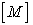, stiffness matrix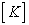and damping matrix [C]. Modal model is represented by a spectral matrix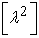(diagonal matrix containing eigenvalues) and modal matrix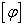(in the columns are eigenvectors). Response model is a result of measurement and consists of FRF matrix (Frequency Response Function)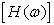.

In theoretical modal analysis we proceed from a physical model (equations of motion) to a modal model (analysis of free oscillation). Modal model is obtained by an analysisof forced harmonicvibration.

In experimental modal analysis we proceed from the response model (properly measured set of FRFs), through modal model (analysis of measured data) up to physical model which is usually not inevitable.

As noted thebase of response models is frequency response function (FRF) given in the form: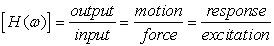(1)

Ifa parameter of response is acceleration, for a single element of frequency response function matrix we can write: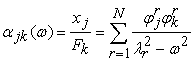(2)

where: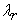eigenvalue of the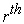mode (natural frequency + modal damping),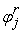theelement of thenatural shapes vector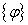,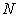number of modes.

Basic assumptions in the modal analysis are linearity, time invariance (stacionarity), reciprocity and observability.

The assumption that the test structure behaves linearly is necessary to achieve the correct FRF measurement. Linearity has two aspects. The first one is that the response is a proportional to applied force. So the larger force is applied to the structure, the larger amplitudes will occur. The second aspect is that we can apply principle of superposition. It means that the resulting response is equal to the sum of the individual responses to each of the forces acting on a structure.

Second assumption is time invariance of the system. It means that modal parameters that we determine are constants.

The next assumption is reciprocity. Reciprocity means that force applied at degree-of-freedom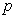causes a response at degree-of-freedom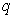that is the same as the response at degree-of-freedomcaused by the same force applied at degree-of-freedom. With respect to FRF there must be valid that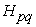is equal to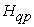.

The last assumption in modal testing is observability. It means that measurement data contain enough information to generate an adequate model of the structure.

The main step in experimental modal analysis is an estimation of modal parameters. There are a number of estimation algorithms. One of them is Rational Fraction Polynomial (RFP) method. This method is based on the theoretical expression used for the FRF which employs the rational fraction formula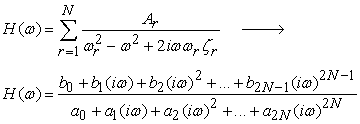(3)

where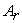is residuum of themode,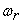is natural frequency of themode,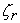is damping ratio of themode,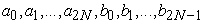are unknown polynomial coefficients which will be sought in the curve-fitting process. These coefficients are clearly related to modal parameters.

An another method designed to provide a global estimation in frequency domain is Complex Mode Indicator Function (CMIF). This method is based on singular value decomposition of frequency response function matrix . This decomposition is defined as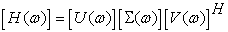(4)

and results in singular values on diagonal of matrix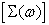left singular vectors contained in matrix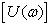and right singular vectors contained in matrix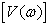, all of which are frequency depended. Singular values are usually plotted as a function of frequency in logarithmic form. The natural frequencies are indicated by the peaks on singular curves. Corresponding left singular vector approximates the mode shape of a given mode and the right singular vector represents modal participation factor . The damping ratio can be estimate from the first singular curve by using the half-power bandwidth method or other advanced technique[1, 3].

### 2. ExperimentsDownload asVeiw figureFigures index
Figure 1. Measuring chain

Object of research was aluminum cantilever beam with dimensions of 960 x 15 x 30 mm. In all measurement, the object was excited by impact hammer Brüel & Kjær type 8206 with plastic tip. This hammer is appropriate to excite small and light structures. Response was measured by laser vibrometer PDV 100 by Polytec company. Parameter of response was velocity. All measurements was performed and evaluate using system PulseTM. Configuration of experiment is shown in Figure 1.

2.1. 1st Experiment

An aim of the first experiment was to measure and compare FRFs measured between two points. In the first case, the object was excited at point 1 and its response was measured at point 2. In the second case, the measurement sequence was reversed (see Figure 2).Download asVeiw figureFigures index
Figure 2. Geometrical models for the 1st experiment.

Sampling frequency was 3.2 kHz and the final FRF is the result of 5 averaging. Measured FRFs are shown in Figure 3.Download asVeiw figureFigures index
Figure 3. FRFs between two points

From the comparison of functions in Figure 3 results relatively high conformity of natural frequency values. The differences are caused by influencing of the structure at excitation and different distances between the beam fixation and the point of excitation in the first and second case.

2.2. 2nd Experiment

An aim of the second experiment was to compare mode shapes measured in the same points but in the opposite measurement sequence. It means that DOFs at which the structure was excited in the first case will be used to measure responses in the second case.

20 DOFs were defined on the upper side of the beam. In the first case, the structure was excited at 18 points (marked by black-green hammer in Figure 4). Response was measured at the remaining two points (marked by red arrow in Figure 4).Download asVeiw figureFigures index
Figure 4. Geometrical model for measurement 1

As can be seen in Figure 5, in the second measurement the measurement sequence was opposite, so the structure was excited in two points and responses were measured in the remaining 18 points.Download asVeiw figureFigures index
Figure 5. Geometry of second measurement

Sampling frequency was 3.2 kHz for both measurements and the measured FRFs are the result of 3 averaging. Modal parameters of the object were estimated in software Pulse Reflex.

Complex Mode Indicator Functions for both measurements are shown in Figure 6 and Figure 7, respectively. Modal parameters were determined by using Polynomial Rational Fraction method. Natural frequencies, damping and complexivity of the individual modes are listed in Table 1 and Table 2.Download asVeiw figureFigures index
Figure 6. CMIF for measurement 1Download asVeiw figureFigures index
Figure 7. CMIF for measurement 2

#### Table 2. Modal parameters of the beam determined based on measurement 2Download asVeiw figureFigures index
Figure 8. First mode shapeof the cantilever beamDownload asVeiw figureFigures index
Figure 9. Second mode shape of the cantilever beam

Obtained mode shapes of the beam are shown in Figure 8 – Figure 11. Shape on the left side of each figure belongs to measurement 1, shape on the right side belongs to measurement 2.Download asVeiw figureFigures index
Figure 10. Third mode shape of the cantilever beamDownload asVeiw figureFigures index
Figure 11. Fourth mode shape of the cantilever beam

Modal Assurance Criterion was used to check mutual collinearity between the mode shapes determined in the first and second measurement. The MAC value between two mode shape vectors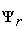and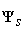is calculated as :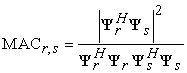(5)

The MAC takes the values from the interval 0 ÷ 1. If MAC is equal to 1, mode shape vectorsandare the same mode shapes. If they are different the MAC value should be low, due to the orthogonality condition of the mode shapes. Cross MAC matrix (see Table 3) shows the degree of conformity between measured modes shapes of the beam. The Table 3 shows that corresponding mode shapes are very similar. On the other hand it is possible to observe a shift of the second and third natural frequency.

### 3. Numerical Modal Analysis

Numerical modal analysis of the beam was performed in NX Nastran. Its aim was to compare experimentally determined modal parameters with the parameters of an idealized model.

Geometrical model was created on the basis of beam dimensions. 10 node tetrahedral elements were used to mesh model. As model material was chosen common aluminium alloy. Natural frequencies determined by FE analysis are shown in Table 4. Mode shapes are shown in Figure12 – Figure 15.

#### Table 4. Natural frequencies (FEA)

Natural frequencies obtained by FE analysis are higher than measured frequencies. It is due to an ideal constraint that is stiffer than real one.Download asVeiw figureFigures index
Figure 12. First mode shapeDownload asVeiw figureFigures index
Figure 13. Second mode shapeDownload asVeiw figureFigures index
Figure 14. Third mode shapeDownload asVeiw figureFigures index
Figure 15. Fourth mode shape

### 4. Conclusion

Experiments described in the paper were focused on the reciprocity in experimental modal analysis. The object of investigation was the cantilever beam made of aluminium. Obtained results showed that the reciprocity of measurement process may be influenced by measurement conditions. It has been shown that a hit of an impact hammer can partly influence the structural properties of an object. A degree of influence depends on its geometry and boundary conditions.

### Acknowledgement

This work was supported by project VEGA 1/0937/12 and KEGA 090TUKE-4/2015.

### References

  F. Trebuňa, F. Šimčák, R. Huňady.: Vibration and modal analysis of mechanical systems, (In Slovak), Typopress, Košice, 2012.In article  A. Bilošová.: Experimental modal analysis, (In Czech), VŠB TU Ostrava.In article  R. J. Allemang, D. L. Brown: A complete review of the complex mode indicator function with applications. International Conference on Noise and Vibration Engineering, ISMA, (2006), 38 pp.In article PubMed  D. J. Ewins: Modal testing – Theory, practice and application. 2. edition, Wiley, (2000).In article  R. Huňady, M.Hagara:Experimental Investigation of Mode Shapes of Symmetric Structures, In: Acta Mechanica Slovaca, Vol 19, No. 3, 2015, 12-17 p.In article  R. J. Allemang: The Modal Assurance Criterion (MAC): Twenty Years of Use and Abuse, Sound & Vibration, August, 2003, pp. 14-21.In article